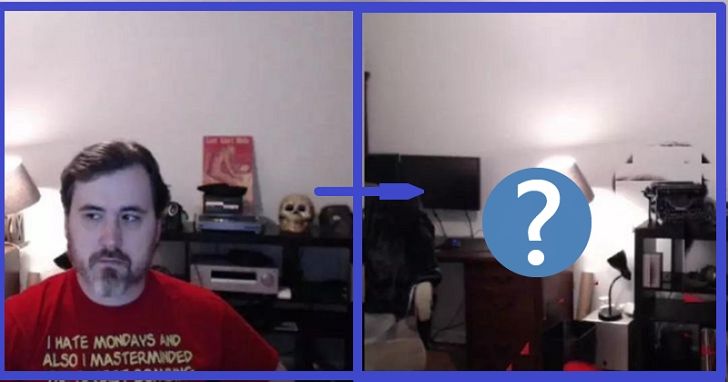### 👉 歡迎加入T客邦telegram  ( https://t.me/TechbangNEWS )https://github.com/jasonmayes/Real-Time-Person-Removal

## 藉助神器TensorFlow.js，200行程式碼實現「隱身」

``// Go through pixels and figure out bounding box of body pixels.  for (let x = 0; x < canvas.width; x++) {    for (let y = 0; y < canvas.height; y++) {      let n = y * canvas.width + x;      // Human pixel found. Update bounds.      if (segmentation.data[n] !== 0) {        if(x < minX) {          minX = x;        }                if(y < minY) {          minY = y;        }        if(x > maxX) {          maxX = x;        }        if(y > maxY) {          maxY = y;        }        foundBody = true;      }    }  }  // Calculate dimensions of bounding box.  var width = maxX - minX;  var height = maxY - minY;``

``// Define scale factor to use to allow for false negatives around this region.  var scale = 1.3;  // Define scaled dimensions.  var newWidth = width * scale;  var newHeight = height * scale;  // Caculate the offset to place new bounding box so scaled from center of current bounding box.  var offsetX = (newWidth - width) / 2;  var offsetY = (newHeight - height) / 2;  var newXMin = minX - offsetX;  var newYMin = minY - offsetY;``

``/ Now loop through update backgound understanding with new data  // if not inside a bounding box.  for (let x = 0; x < canvas.width; x++) {    for (let y = 0; y < canvas.height; y++) {      // If outside bounding box and we found a body, update background.      if (foundBody && (x < newXMin || x > newXMin + newWidth) || ( y < newYMin || y > newYMin + newHeight)) {        // Convert xy co-ords to array offset.        let n = y * canvas.width + x;        data[n * 4] = dataL[n * 4];        data[n * 4 + 1] = dataL[n * 4 + 1];        data[n * 4 + 2] = dataL[n * 4 + 2];        data[n * 4 + 3] = 255;      } else if (!foundBody) {        // No body found at all, update all pixels.        let n = y * canvas.width + x;        data[n * 4] = dataL[n * 4];        data[n * 4 + 1] = dataL[n * 4 + 1];        data[n * 4 + 2] = dataL[n * 4 + 2];        data[n * 4 + 3] = 255;      }    }  }  ctx.putImageData(imageData, 0, 0);``

https://codepen.io/jasonmayes/

## TensorFlow.js能做的遠不止讓你「消失」

TensorFlow.js是一個使用JavaScript進行機器學習開發的庫，允許使用JavaScript進行機器學習模型的開發，並可以直接在瀏覽器或Node.js中使用機器學習模型。

``const net = await bodyPix.load({ architecture: 'MobileNetV1', outputStride: 16, multiplier: 0.75, quantBytes: 2});``## 技術比創造力多一點點的15年老程式設計師